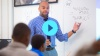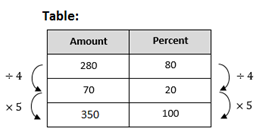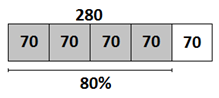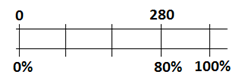# Percent and Scaling

Students expand on and apply their understanding of percentages by studying percent increase and decrease, percent applications such as tax and simple interest, and scaled geometric drawings.

Math

Unit 5

## Unit Summary

In Unit 5, 7th grade students use their understanding of ratios and proportions from Unit 1 to solve various real-world applications involving percentages and scale drawings. They begin the unit by studying the relationship between percent, part, and whole using familiar strategies from 6th grade as well as new strategies from this year, such as proportions. Students then investigate how percentages can be used to represent increases and decreases in quantities. They use visual diagrams to model these situations and to understand how 100% plus 20% of a value is the same as 120% of that value (MP.2). Students continue to build fluency with percent problems as they solve real-world problems such as calculating purchase totals while considering discounts and tax. Lastly, students study scale drawings and learn how a scale can be used to create scale copies of large measurements such as maps or floor plans. They apply proportional reasoning and strategically use tools to re-create scale drawings or find actual measures from scale drawings (MP.5). Throughout this unit, students use skills and concepts they already have to make sense of and reason through new problems (MP.1), including continuing to work with a range of rational numbers.

In 6th grade, students learned several strategies to solve ratio and rate problems, including tables, tape diagrams, double number lines, and equations. They also defined percent as a rate per 100 and solved percent problems to find the whole, part, or percent. These standards are foundational to this 7th grade unit, and the first four lessons in this unit incorporate these concepts and skills.

In 8th Grade Math, students will refine their understanding of scale and scale drawings when they study dilations in their transformations unit. They will define similar figures and use dilations and other transformations to prove that two images are similar or scale drawings of one another.

Pacing: 23 instructional days (19 lessons, 3 flex days, 1 assessment day)

Fishtank Plus for Math

Unlock features to optimize your prep time, plan engaging lessons, and monitor student progress.## Assessment

The following assessments accompany Unit 5.

### Pre-Unit

Have students complete the Pre-Unit Assessment and Pre-Unit Student Self-Assessment before starting the unit. Use the Pre-Unit Assessment Analysis Guide to identify gaps in foundational understanding and map out a plan for learning acceleration throughout the unit.

### Mid-Unit

Have students complete the Mid-Unit Assessment.

### Post-Unit

Use the resources below to assess student understanding of the unit content and action plan for future units.

Expanded Assessment Package

Use student data to drive your planning with an expanded suite of unit assessments to help gauge students’ facility with foundational skills and concepts, as well as their progress with unit content.

## Unit Prep

### Intellectual Prep

Unit Launch

Prepare to teach this unit by immersing yourself in the standards, big ideas, and connections to prior and future content. Unit Launches include a series of short videos, targeted readings, and opportunities for action planning.#### Internalization of Standards via the Post-Unit Assessment

• Take the Post-Unit Assessment. Annotate for:
• Standards that each question aligns to
• Strategies and representations used in daily lessons
• Relationship to Essential Questions of unit
• Lesson(s) that Assessment points to

#### Internalization of Trajectory of Unit

• Read and annotate the Unit Summary.
• Notice the progression of concepts through the unit using the Lesson Map.
• Essential Understandings
• Connection to Post-Unit Assessment questions
• Identify key opportunities to engage students in academic discourse. Read through our Teacher Tool on Academic Discourse and refer back to it throughout the unit.

#### Unit-Specific Intellectual Prep

• Read the following Progressions for the Common Core State Standards in Mathematics for the standards relevant to this unit
• Read the following table that includes models used throughout the unit
 Model Example Table 280 students attend a carnival, representing 80% of the school.Tape diagram 280 students attend a carnival, representing 80% of the school.Double number line 280 students attend a carnival, representing 80% of the school.Proportion 280 students attend a carnival, representing 80% of the school. $$\frac{280}{x}=\frac{80}{100}$$ $$x=350$$ Percent equation 280 students attend a carnival, representing 80% of the school.  $$280=0.80x$$ $$x=280/0.80$$ $$x=350$$

### Essential Understandings

• Percentages can be used to understand the relationship between parts of quantities and the whole quantity. Percentages can also be used to understand how quantities change in relation to their starting values. As a result, we can use percentages to model many real-world applications such as price changes and simple interest.
• Scale drawings are proportional to one another by a measure called the scale factor.
• Scales, such as “1 inch represents 10 miles,” enable us to draw and work with large or small objects and measures at scale, for example maps and floor plans.

### Materials

• String (1 per student) — A different flexible measuring tool also works here.
• Graph Paper (2-3 sheets per student)
• Blank Paper (2-3 sheets per student)
• Ruler (1 per student)

To see all the materials needed for this course, view our 7th Grade Course Material Overview.

### Vocabulary

commission

corresponding

discount

markup/markdown

measurement error

percent increase/percent decrease

percent error

scale factor

scale

scale image/drawing

simple interest

tax

tip

To see all the vocabulary for Unit 5, view our 7th Grade Vocabulary Glossary.

## Lesson Map

Topic A: Percent, Part, and Whole

Topic B: Percent Increase and Decrease

Topic C: Percent Applications

Topic D: Scale Drawings

## Common Core Standards

Key

Major Cluster

Supporting Cluster

### Core Standards

#### Expressions and Equations

• 7.EE.A.2 — Understand that rewriting an expression in different forms in a problem context can shed light on the problem and how the quantities in it are related. For example, a + 0.05a = 1.05a means that "increase by 5%" is the same as "multiply by 1.05."
• 7.EE.B.3 — Solve multi-step real-life and mathematical problems posed with positive and negative rational numbers in any form (whole numbers, fractions, and decimals), using tools strategically. Apply properties of operations to calculate with numbers in any form; convert between forms as appropriate; and assess the reasonableness of answers using mental computation and estimation strategies. For example: If a woman making $25 an hour gets a 10% raise, she will make an additional 1/10 of her salary an hour, or$2.50, for a new salary of \$27.50. If you want to place a towel bar 9 3/4 inches long in the center of a door that is 27 1/2 inches wide, you will need to place the bar about 9 inches from each edge; this estimate can be used as a check on the exact computation.

#### Geometry

• 7.G.A.1 — Solve problems involving scale drawings of geometric figures, including computing actual lengths and areas from a scale drawing and reproducing a scale drawing at a different scale.

#### Ratios and Proportional Relationships

• 7.RP.A.3 — Use proportional relationships to solve multistep ratio and percent problems. Examples: simple interest, tax, markups and markdowns, gratuities and commissions, fees, percent increase and decrease, percent error.

#### The Number System

• 7.NS.A.3 — Solve real-world and mathematical problems involving the four operations with rational numbers. Computations with rational numbers extend the rules for manipulating fractions to complex fractions.

• 6.G.A.1

• 5.NF.B.5
• 5.NF.B.5.A
• 5.NF.B.5.B

• 6.RP.A.3
• 6.RP.A.3.C
• 7.RP.A.2

• 8.G.A.2
• 8.G.A.4

### Standards for Mathematical Practice

• CCSS.MATH.PRACTICE.MP1 — Make sense of problems and persevere in solving them.

• CCSS.MATH.PRACTICE.MP2 — Reason abstractly and quantitatively.

• CCSS.MATH.PRACTICE.MP3 — Construct viable arguments and critique the reasoning of others.

• CCSS.MATH.PRACTICE.MP4 — Model with mathematics.

• CCSS.MATH.PRACTICE.MP5 — Use appropriate tools strategically.

• CCSS.MATH.PRACTICE.MP6 — Attend to precision.

• CCSS.MATH.PRACTICE.MP7 — Look for and make use of structure.

• CCSS.MATH.PRACTICE.MP8 — Look for and express regularity in repeated reasoning.

Unit 4

Equations and Inequalities

Unit 6

Geometry

## Request a Demo

See all of the features of Fishtank in action and begin the conversation about adoption.

Yes

No

### Any other information you would like to provide about your school?